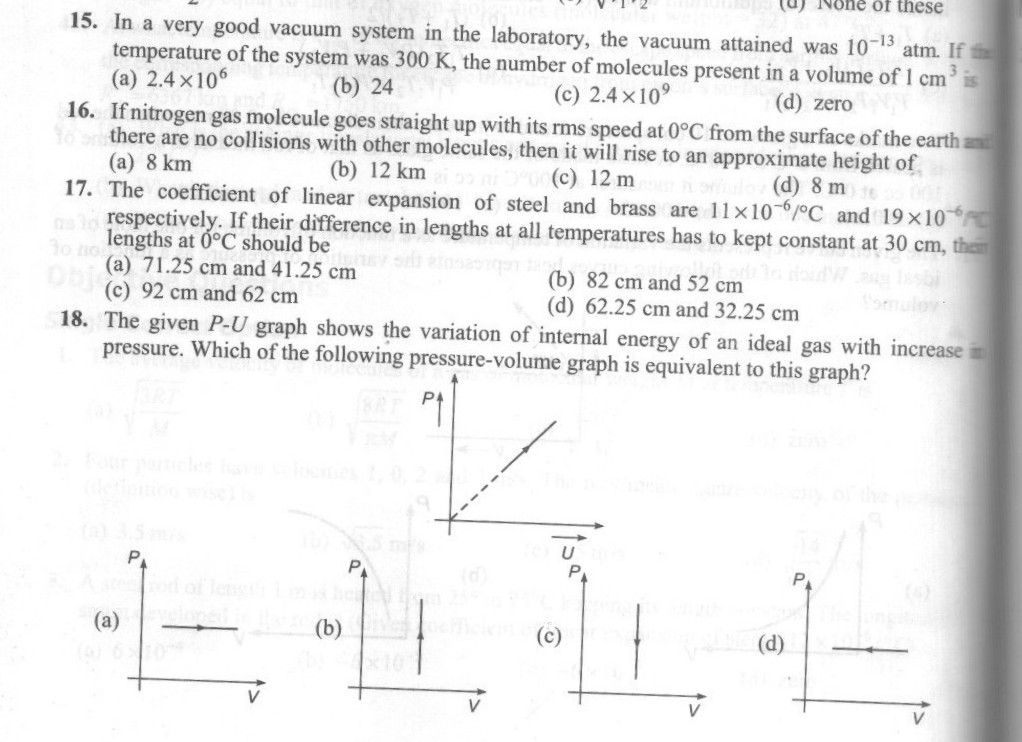# Therma Physicsall question ..pl show the working

•Anurag Ghosh ·

15.Use PV=nRT to find the no. of moles....and then no. of molecules=n*6.02*10^23

16.Apply energy conservation,1/2*m*Vrms^2=mgH,its 12m..may be the answer is wrong.

17.I am not getting it now,will try later on..

18.(b)....U=n*Cv*del.T.....which is at constant volume,so eliminate a and d first,then in b pressure is increasing,so b is correct..

•17. Consider the lengths of the steel and brass rods at 0Â°C to be ls and lb respectively. Then difference of their lengths at any temperature is equal to ls - lb = 30 cm. The answer is coming as (A).

•Sahil Jain ·

16. vrms= âˆš3rt/m =
h = v2/2g = 3rt/2mg â‰ˆ 12.11 m but the ans is 12 km ???

•Himanshu Giria ·

1.) (a) 2.4*10^6